# AP Chemistry : Radioactive Decay and Nuclear Chemistry

## Example Questions

### Example Question #1 : Radioactive Decay And Nuclear Chemistry

What kind of radiation has no charge or mass?

alpha

delta

gamma

beta

gamma

Explanation:

This is the definition of gamma radiation.

### Example Question #1 : Help With Radioactive Decay

Consider the following isotope of thorium: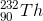What is the identity of the product following three alpha decay reactions?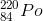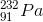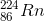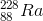Explanation:

During alpha decay, an element emits a helium nucleus with 2 neutrons and 2 protons. Thus, the atomic mass of the new element is decreased by four, and the atomic number is decreased by two.

Three subsequent alpha decays result in a new element with an atomic mass of 232 - 3(4) = 220, and a new atomic number of 90 - 3(2) = 84.

Using the periodic table, we find the element with this atomic number is polonium (Po).

### Example Question #3 : Radioactive Decay And Nuclear Chemistry

Consider the following isotope: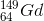What is the identity of the product after the following series of decay reactions?

alpha decay, alpha decay, electron emission, positron emission, positron emission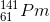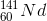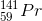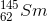Explanation:

In alpha decay, a helium nucleus is emitted, and thus the isotope loses 2 protons and 2 neutrons.

In electron emission, a neutron in the nucleus is converted into a proton and an emitted electron.

In positron emission, a proton in the nucleus is converted into a neutron and an emitted positron.

The given isotope will lose 4 protons and 4 neutrons via alpha emission, gain 1 proton and lose 1 neutron via electron emission, and lose 2 protons and gain 2 neutrons via positron emission. The result is a loss of 5 protons and 8 mass units.

Accounting for the changes in atomic mass and number, we find that the final element is 141-praseodymium.

### Example Question #1 : Radioactive Decay And Nuclear Chemistry

Uranium-238 undergoes alpha decay. Which nucleus is formed as a result of this decay?

Uranium-238

Thorium-234

He-4

Uranium-234

Thorium-234

Explanation:

In alpha decay, an element loses the equivalent of a helium nucleus, or 2 protons and 2 neutrons. Uranium-238 has an atomic number of 92 and a mass of 238 units, so the product of alpha decay will have an atomic number of 90 and a mass of 234 units. This corresponds to thorium-234.

### Example Question #9 : Elements, Ions, And Isotopes

Am-242 undergoes beta-decay at a half-life of 16 hours. If a chemist starts with a sample of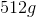of Am-242 and allows it to undergo beta-decay, how much of the original sample remains after 4 days?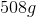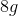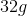Explanation:

With each half-life, the amount of Am-242 is halved. 4 days is 96 hours, which is 6 half-lives. For half-life questions use the formula: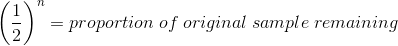Whereis the number of half lives.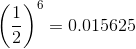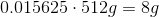Thusof Am-242 is left after 4 days.

### Example Question #10 : Elements, Ions, And Isotopes

An alpha particle can also be written as which of the following?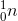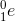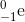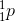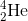Explanation:

An alpha particle can be written as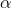or. The latter is used frequently in nuclear physics because it is more helpful when calculating resulting nuclei after radioactive decay. In alpha emission, the nucleus loses two protons and two neutrons.

### Example Question #1 : Radioactive Decay And Nuclear Chemistry

The isotope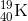of potassium is used to date geological materials. One of the decay reactions this isotope undergoes is: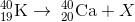In order for the nuclear reaction to be balancedcan be:

an electron

either an electro or β radiation

a proton

either an electro or β radiation

Explanation:

A beam of electrons is known also as β radiation. An electron has atomic number ofand zero mass number. Hence, the reaction can be written: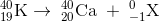Where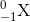can be either, an electron or β radiation. The mass number is balanced because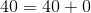and the atomic number is balanced because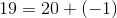. This kind of nuclear reactions are called beta decay reactions.

### Example Question #2 : Radioactive Decay And Nuclear Chemistry

Suppose that a radioactive isotope whose half-life is equal to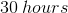is allowed to decay. After waiting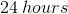, how much of this compound would be expected to exist compared to the initial amount?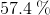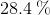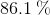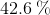Explanation:

In order to answer this question, we'll need to employ the equation for first-order decay. The reason we know that this is a first order decay process is because we are told that the compound in question is radioactive. It's important to recall that all radioactive decay processes occur via a first order decay mechanism.

Before jumping into the math, let's first make a quick ballpark prediction of where our answer should be. We know that the half-life for any compound is the amount of time it takes for half of that compound to decay. Furthermore, we're given the value for a compound's half-life. Since the amount of time that has passed in the question is less than the half-life, we would expect to still have over half of the compound left. Thus, our answer should be above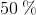.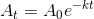Where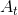is the amount of compound that exists at a specified time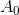is the amount of compound that exists initiallyis the decay constant for the compoundis the specified amount of time that has passed

To solve for the fraction of compound that exists at a given time relative to the initial amount, we can make the following changes to the above equation.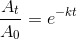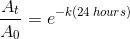Thus far, we have everything we need except for the decay constant. However, as long as we know the half life of the compound, we can calculate the decay constant using the following formula that applies to all first order reactions.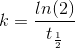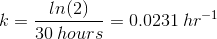Notice that the above decay constant has units of inverse-time. This is what we would expect, since all first order reactions have rate constants with these units.

Now, we are ready to plug in the value we calculated for the decay constant into the rate equation to find our answer.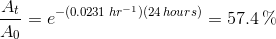Going back to the quick prediction we made, we can see that this calculated value agrees with our prediction.